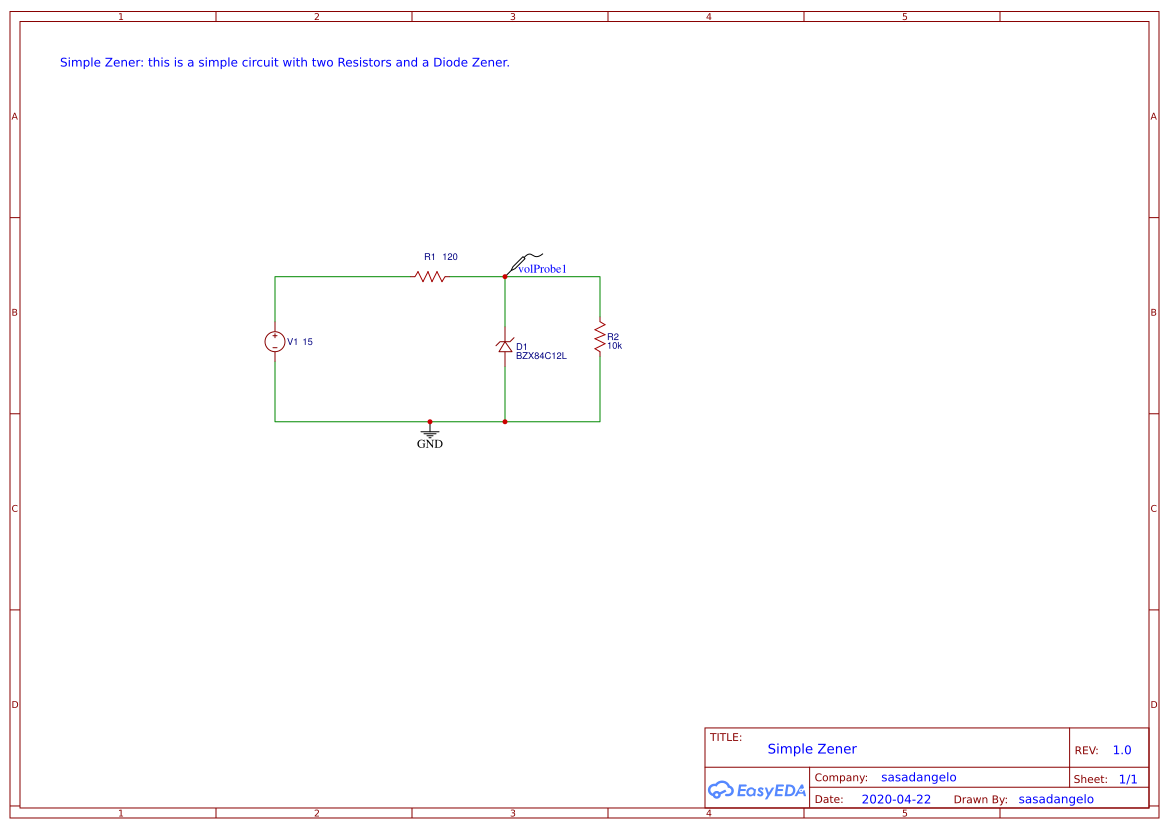Public Domain

##### Tags
Recommend Projects

# Simple Zener

2 months ago 70
• Description
• Documents
• BOM
• Attachments
• Members

## Description

This is a simple circuit with a 12 V Diode Zener, a 120-ohm Resistor and a 15V battery. The resistor R1 is calculated with the formula:

R1=(Vcc-Vz)/Iz

Where Vcc is the 15V battery voltage, Vz is the breakdown voltage, and Iz is the current absorbed by the Diode. By design, you should assume Iz=25mA. According to this information, R1 is:

R1=(15V-12V)/0.025A=120 Ohm

The formula above makes sense only if we assume I1 (current passing through the resistor R1) is almost equal to Iz (current passing through the diode Zener). This is true if and only I2 (current passing through R2) is negligible. This usually is true if the value of resistor R2 is high (i.e. 10KOhm, 100Khm, and so on).

I verified with EasyEda that the 12V voltage is stable if we resistor with a minimum value of 300 Ohm, below this value the Diode stop working.

## Simple Zener Circuit## BOM

ID Name Designator Footprint Quantity
1 15 V1 HDR1X2 1
2 10k R2 R0201 1
3 120 R1 AXIAL-0.3 1
4 BZX84C12L D1 SMB 1

## Attachments

None
Success
The owner does not allow comments in this project now